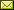## Distributed Queuing in Dynamic Networks

 Gokarna Sharma (Louisiana State University) Costas Busch (Louisiana State University)

 We consider the problem of forming a distributed queue in the adversarial dynamic network model of Kuhn, Lynch, and Oshman (STOC 2010) in which the network topology changes from round to round but the network stays connected. This is a synchronous model in which network nodes are assumed to be fixed, the communication links for each round are chosen by an adversary, and nodes do not know who their neighbors are for the current round before they broadcast their messages. Queue requests may arrive over rounds at arbitrary nodes and the goal is to eventually enqueue them in a distributed queue. We present two algorithms that give a total distributed ordering of queue requests in this model. We measure the performance of our algorithms through round complexity, which is the total number of rounds needed to solve the distributed queuing problem. We show that in 1-interval connected graphs, where the communication links change arbitrarily between every round, it is possible to solve the distributed queueing problem in O(nk) rounds using O(log n) size messages, where n is the number of nodes in the network and k <= n is the number of queue requests. Further, we show that for more stable graphs, e.g. T-interval connected graphs where the communication links change in every T rounds, the distributed queuing problem can be solved in O(n+ (nk/min(alpha,T))) rounds using the same O(log n) size messages, where alpha > 0 is the concurrency level parameter that captures the minimum number of active queue requests in the system in any round. These results hold in any arbitrary (sequential, one-shot concurrent, or dynamic) arrival of k queue requests in the system. Moreover, our algorithms ensure correctness in the sense that each queue request is eventually enqueued in the distributed queue after it is issued and each queue request is enqueued exactly once. We also provide an impossibility result for this distributed queuing problem in this model. To the best of our knowledge, these are the first solutions to the distributed queuing problem in adversarial dynamic networks.

In Keren Censor-Hillel and Valerie King: Proceedings Ninth International Workshop on Foundations of Mobile Computing (FOMC 2013), Jerusalem, Israel, October 17-18, 2013, Electronic Proceedings in Theoretical Computer Science 132, pp. 1–19.
Published: 17th October 2013.

 ArXived at: https://dx.doi.org/10.4204/EPTCS.132.1 bibtex PDF
References in reconstructed bibtex, XML and HTML format (approximated).
 Comments and questions to:eptcs@eptcs.org For website issues:webmaster@eptcs.org